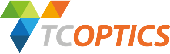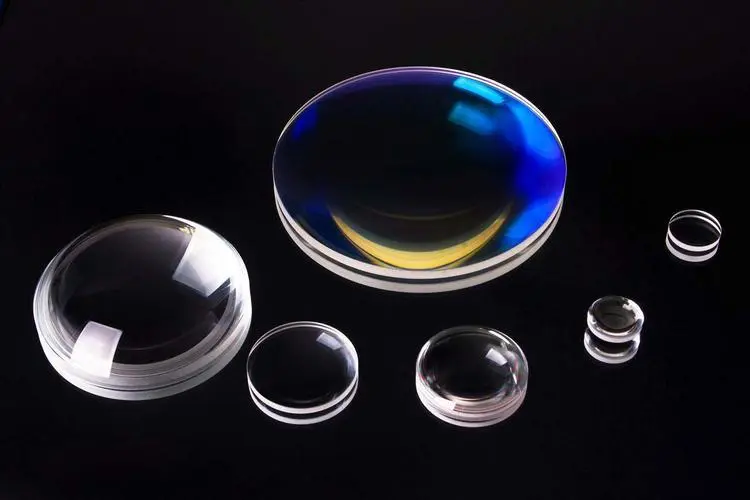﻿ The Classifications and Working Principles of Optical Lenses - TianCheng Optics CO., Ltd.# The Classifications and Working Principles of Optical LensesOptical lenses are made according to the law of light refraction. A lens is an optical element made of transparent materials (such as glass, crystal, etc.). The lens is a refracting lens, and its refractive surface is a transparent body with two spherical surfaces (part of the spherical surface), or one spherical surface (part of the spherical surface) and one plane. The images it forms have real and virtual images.

Lenses can generally be divided into two categories: plano convex and plano concave lenses. The center part is thicker than the edge part is called a convex lens, there are three kinds of biconvex, plano-convex, and concave-convex; the center part is thinner than the edge part is called a concave lens, including bi-concave, plano-concave, and convex-concave. Lens classification: Convex lens has the function of condensing light, so it is also called "converging lens" and "positive lens" (which can be used for hyperopia and reading glasses). Such lenses can be divided into:

a. Double-concave lens-a lens on both sides;

b. Plano-concave lens-a lens with a concave side and a flat side;

c. Concave convex lens-a lens with one convex and one concave lens.

A thin lens is a lens in which the thickness of the central part is larger than the radius of curvature of the two surfaces. In the early days, the camera was only equipped with a convex lens, so it was called a "single lens." With the development of science and technology, modern lenses have several concave convex lenses with different forms and functions to form a convergent lens, called a "compound lens." The concave lens in the compound lens functions to correct various aberrations. Optical glass has high transparency, purity, colorlessness, uniform texture, and good refractive power, so it is the main raw material for lens production.

## Ⅰ. Optical glasses with different chemical compositions and refractive indices

1. Flint glass-lead oxide is added to the glass composition to increase the refractive index (1.8804);

2. Crown glass-made by adding sodium oxide and calcium oxide to the glass composition to reduce its refractive index (the refractive index of barium crown glass is 1.7055);

3. Lanthanum crown glass-a variety discovered in recent years, it has the excellent characteristics of high refractive index and low dispersion rate, which provides conditions for the creation of large-aperture advanced lenses.

The principle of lens imaging is that a glass or plastic component used in lamps can change the direction of light or control the distribution of light. Lens is the most basic optical element that composes the microscope optical system. The objective lens, eyepiece, condenser and other components are composed of single and multiple lenses. According to their different shapes, they can be divided into two categories: convex lens (positive lens) and concave lens (negative lens). When a beam of light parallel to the main optical axis passes through the convex lens and intersects at a point, this point is called the "focus", and the plane passing through the focus and perpendicular to the optical axis is called the "focal plane". There are two focal points. The focal point in the object space is called the "object focal point", and the focal plane is called the "object focal plane"; conversely, the focal point in the image space is called the "image focal point". The focal plane at the position is called the "image square focal plane". After the light passes through the concave lens, it becomes an erect virtual image, while the convex lens becomes an inverted real image. The real image can be displayed on the screen, but the virtual image can't.

## Ⅱ. The imaging law of lens and convex lens

1. The lens is represented by the lens symbol (a line segment has two V-shaped signs at both ends), draw the main optical axis, mark the optical center, and the focal point. According to the two refracted rays of the three special rays of the lens, the light is generally used. The intersection point of the central light and the light parallel to the main optical axis is better, and the characteristics of the image formed by the lens (such as virtual reality, size, front and back, etc.) can be obtained.

2. When the lens is imaging, all the light shining on the lens from each point on the object is imaged in the same position, blocking a part, and does not affect the imaging of other light rays to the lens, so the complete image can still be seen. However, as the light hitting the image is reduced, the brightness of the image on the screen will become darker.

3. Convex lens imaging law:

(1) A requirement that the convex lens needs to meet to form a real image is (u>f).

(2) Conjugate imaging means that the size of the object distance and the image distance can be interchanged. In the two cases, it becomes an enlarged and reduced inverted real image.

4. Looking through a convex lens at a timepiece other than the double focal length, the image of the second hand still rotates clockwise, because it is an inverted real image, and it is still in the normal direction when viewed backwards, so it still rotates clockwise.TCOPTICS, Your Professional Optics Manufacturing Company
NEWS
• 18686471870

• 86-431-89851801